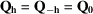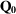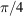International Tables for Crystallography (2006). Vol. B, ch. 5.3, pp. 557-569   | 1 | 2 | https://doi.org/10.1107/97809553602060000571

## Contents

• 5.3. Dynamical theory of neutron diffraction  (pp. 557-569)
• 5.3.1. Introduction  (p. 557) | html | pdf |
• 5.3.2. Comparison between X-rays and neutrons with spin neglected  (pp. 557-558) | html | pdf |
• 5.3.2.1. The neutron and its interactions  (p. 557) | html | pdf |
• 5.3.2.2. Scattering lengths and refractive index  (pp. 557-558) | html | pdf |
• 5.3.2.3. Absorption  (p. 558) | html | pdf |
• 5.3.2.4. Differences between neutron and X-ray scattering  (p. 558) | html | pdf |
• 5.3.2.5. Translating X-ray dynamical theory into the neutron case  (p. 558) | html | pdf |
• 5.3.3. Neutron spin, and diffraction by perfect magnetic crystals  (pp. 558-561) | html | pdf |
• 5.3.3.1. Polarization of a neutron beam and the Larmor precession in a uniform magnetic field  (pp. 558-559) | html | pdf |
• 5.3.3.2. Magnetic scattering by a single ion having unpaired electrons  (pp. 559-560) | html | pdf |
• 5.3.3.3. Dynamical theory in the case of perfect ferromagnetic or collinear ferrimagnetic crystals  (pp. 560-561) | html | pdf |
• 5.3.3.4. The dynamical theory in the case of perfect collinear antiferromagnetic crystals  (p. 561) | html | pdf |
• 5.3.3.5. The flipping ratio  (p. 561) | html | pdf |
• 5.3.4. Extinction in neutron diffraction (non-magnetic case)  (pp. 561-562) | html | pdf |
• 5.3.5. Effect of external fields on neutron scattering by perfect crystals  (p. 562) | html | pdf |
• 5.3.6. Experimental tests of the dynamical theory of neutron scattering  (pp. 562-563) | html | pdf |
• 5.3.7. Applications of the dynamical theory of neutron scattering  (pp. 563-564) | html | pdf |
• 5.3.7.1. Neutron optics  (p. 563) | html | pdf |
• 5.3.7.2. Measurement of scattering lengths by Pendellösung effects  (p. 563) | html | pdf |
• 5.3.7.3. Neutron interferometry  (pp. 563-564) | html | pdf |
• 5.3.7.4. Neutron diffraction topography and other imaging methods  (p. 564) | html | pdf |
• References | html | pdf |
• Figures
• Fig. 5.3.3.1. Schematic plot of the two-beam dispersion surface in the case of a purely magnetic reflection such thatand that the angle betweenandis equal to(p. 560) | html | pdf |